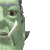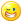## Welcome to the Second Life Forums Archive

These forums are CLOSED. Please visit the new forums HERE

# Binary Math

MC Seattle
Registered User
Join date: 3 Apr 2006
Posts: 63
04-14-2006 03:36
CODE
`// LSL Binary Arithmetic// (Anti-)Proof of concept//// The largest numbers LSL supports are 32-bit signed // integers, which limits llModPow to 16-bit exponents, // therefore limiting key length in RSA encryption and // Diffie-Hellman exchanges to 16-bit keys. To get // around this limitation I started writing a binary // arithmetic library which would allow arbitrary size // numbers in ModPow functions, bringing LSL up to // par with the strength of PHP libraries like bcmath and // gmp. Unfortunately, the speed of LSL makes this a // laughable experience. Multiplying the two 32-bit // test numbers takes over 30 seconds in my tests. I // guess 64-bit exponents are out of the question...//     (What was I thinking?)// Anyways instead of throwing the code away I'm // archiving it here in case anyone else gets the wild // idea to try something similar. Addition and subtraction // can be done very quickly if someone has a use for those, // and this could probably be optimized by using strings // instead of lists. Only addition and multiplication are // implemented in this version, but from my testing they // are both very robust. Unless you start passing non-// binary data in of course. // Some random test values, 3816682369 and 2305728161list number_one = [1,1,1,0,0,0,1,1,0,1,1,1,1,1,0,1,1,1,1,1,0,0,1,1,1,0,0,0,0,0,0,1];list number_two = [1,0,0,0,1,0,0,1,0,1,1,0,1,1,1,0,1,0,0,1,1,1,1,0,1,0,1,0,0,0,0,1];print_binary(list number){    integer i;    string output;        for (i = 0; i < llGetListLength(number); i++) {        output += llList2String(number, i);    }        llSay(0, output);}list reverse(list number){    list new;    integer i;        for (i = llGetListLength(number) - 1; i >= 0; i--) {        new += llList2List(number, i, i);    }        return new;}list add(list first, list second){    list answer = [];    list temp = [];    integer i;    integer f;    integer s;    integer carry = FALSE;    integer diff = llGetListLength(first) - llGetListLength(second);    // Pad the one of the numbers with leading zeroes if necessary       if (diff < 0) {        for (i = 0; i < llAbs(diff); i++) {            temp += ;        }                first = temp + first;    } else if (diff > 0) {        for (i = 0; i < diff; i++) {            temp += ;        }                second = temp + second;    }        // Addition loop    for (i = llGetListLength(first) - 1; i >= 0; i--) {        f = llList2Integer(first, i);        s = llList2Integer(second, i);        if (f && s && carry) {            carry = TRUE;            answer += ;        } else if (f && s || ((f || s) && carry)) {            carry = TRUE;            answer += ;        } else {            if (f || s || carry) {                answer += ;            } else {                answer += ;            }                        carry = FALSE;        }    }        if (carry) {        answer += ;    }        return reverse(answer);}list multiply(list multiplicand, list multiplier){    integer i;    integer j;    list partial_sum = ;    list product;    integer length = llGetListLength(multiplier);        for (i = length - 1; i >= 0; i--) {        product = [];                if (llList2Integer(multiplier, i)) {            // Build the right-side zero padding for the product            for (j = 0; j < (length - i) - 1; j++) {                product += ;            }                        product = multiplicand + product;            partial_sum = add(partial_sum, product);        }    }        return partial_sum;}default{    state_entry()    {    }    touch_start(integer total_number)    {        list answer;                llSay(0, "Free memory: " + (string)llGetFreeMemory());                llSay(0, "Binary addition test");        print_binary(number_one);        print_binary(number_two);        llSay(0, "--------------------------------");        answer = add(number_one, number_two);        print_binary(answer);                llSay(0, "Binary multiplication test");        print_binary(number_one);        print_binary(number_two);        llSay(0, "--------------------------------");        answer = multiply(number_one, number_two);        print_binary(answer);                llSay(0, "Free memory: " + (string)llGetFreeMemory());    }}`
Nada Epoch
The LibrarianJoin date: 4 Nov 2002
Posts: 1,423
Discussion Thread
04-14-2006 09:02
/54/3a/100261/1.html
_____________________
i've got nothing.